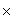Name: David, an elementary school teacher I am preparing a student for a math contest.  A prep question is, "How many positive integers less than or equal to 250 are relatively prime with 250?"  I do not know the answer or how to figure it out. Help! Hi David. Two numbers are relatively prime (this is also called "co-prime") if they share no prime factors. So let's take a look at the prime factorisation of 250: Prime factorisation: 250 = 253 So any number that divides 5 or 2 is not co-prime with 250. That rules out all the even numbers as well as all numbers ending in 5 or 0. So how many are left? There are 125 odd numbers under 250 and 1/5 of all these odd numbers ends in 5 (the zeros were already elimated because they are even). That means your answer is 4/5(125). There's a bit of a question about whether you should consider the value of "1" itself. It isn't a prime number (the first prime is 2), but it shares no prime factors with 250, either. So depending on your definition, there may be 79 or 80 in all. Hope this helps! Stephen La Rocque.